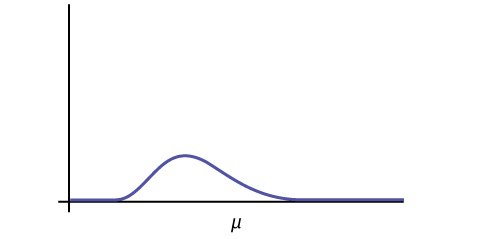# 8.2: Facts About the Chi-Square Distribution

The notation for the chi-square distribution is:

$\chi \sim \chi^{2}_{df}$

where $$df =$$ degrees of freedom which depends on how chi-square is being used. (If you want to practice calculating chi-square probabilities then use $$df = n - 1$$. The degrees of freedom for the three major uses are each calculated differently.)

For the $$\chi^{2}$$ distribution, the population mean is $$\mu = df$$ and the population standard deviation is

$\sigma = \sqrt{2(df)}.$

The random variable is shown as $$\chi^{2}$$, but may be any upper case letter. The random variable for a chi-square distribution with $$k$$ degrees of freedom is the sum of $$k$$ independent, squared standard normal variables.

$\chi^{2} = (Z_{1})^{2} + ... + (Z_{k})^{2}$

1. The curve is nonsymmetrical and skewed to the right.
2. There is a different chi-square curve for each $$df$$.Figure $$\PageIndex{1}$$

3. The test statistic for any test is always greater than or equal to zero.
4. When $$df > 90$$, the chi-square curve approximates the normal distribution. For $$\chi \sim \chi^{2}_{1,000}$$ the mean, $$\mu = df = 1,000$$ and the standard deviation, $$\mu = \sqrt{2(1,000)}$$. Therefore, $$X \sim N(1,000, 44.7)$$, approximately.
5. The mean, $$\mu$$, is located just to the right of the peak.Figure $$\PageIndex{2}$$